# Eval y=f(a,x) VI

#### NI Formula Parsing VIs

Eval y=f(a,x) VI

Owning Palette: 1D & 2D Evaluation VIs

Installed With: Full Development System

A generalized version of the Eval y=f(x) VI with the possibility of adding some parameters into the formula.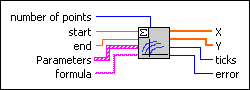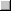Place on the block diagramFind on the Functions palette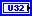number of points is the number of all calculated points. The independent variable is split into equidistant subpoints. The default is 10.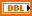start is the start point of the interval. The default is 0.0.end is the end point of the interval. The default is 1.0.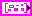Parameters is an array of clusters describing the parameters.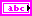name of the parameter that uses the conventions of the Formula Parsing VIs.value is the user-defined value of the parameter.formula is a string describing the function under investigation. The formula can contain any number of valid variables.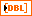X is the array of equidistant points between start and end.Y is the function values at the points X.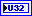ticks is the time in milliseconds to analyze the formula and to produce the X and the Y array.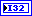error returns any error or warning from the VI. You can wire error to the Error Cluster From Error Code VI to convert the error code or warning into an error cluster.